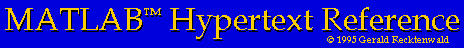# Programming MATLAB

Soon after you become accustomed to using MATLAB interactively you will probably grow tired of typing the same sequences of commands in order to perform routine tasks. When this happens you will be ready to start programming in MATLAB.

MATLAB ``programs'' are sequences of MATLAB commands saved in plain text files. MATLAB requires that these files have the extension ``.m''. As a result MATLAB programs are often referred to as m-files.

There are two types of MATLAB programs. These are scripts and functions.

## Scripts

• Scripts versus Functions
• Creating a script m-file
• Recording a script with the diary command
• Using (and abusing) scripts
• Side effects on the MATLAB workspace

## MATLAB Functions -- Intermediate Features

• Functions versus Scripts
• Creating a function m-file
• Structure of a MATLAB function
• Input and Output parameters
• Comment statements
• Function prologue -- providing help
• Calling MATLAB Functions
• Local Variables
• Flow Control
• if constructs
• for constructs
• while constructs

## MATLAB Functions -- Intermediate Features

• Variable numbers of input and output parameters
• Global variables

## Improving the Speed of MATLAB Calculations

• Using vector operations instead loops
• Pre-allocating memory for vectors and matrices